Скачать презентацию Data Modeling Patrice Koehl Department of Biological Sciences

45d035ba6e35f5a7e6399ecbda710f89.ppt

• Количество слайдов: 25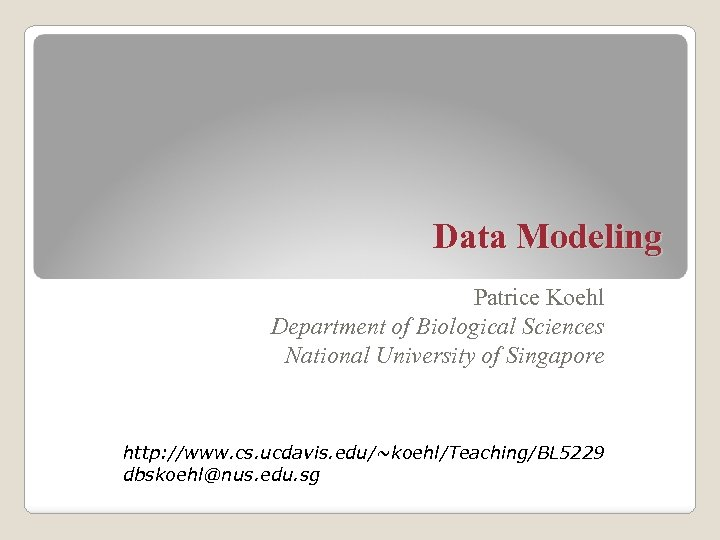Data Modeling Patrice Koehl Department of Biological Sciences National University of Singapore http: //www. cs. ucdavis. edu/~koehl/Teaching/BL 5229 [email protected] edu. sg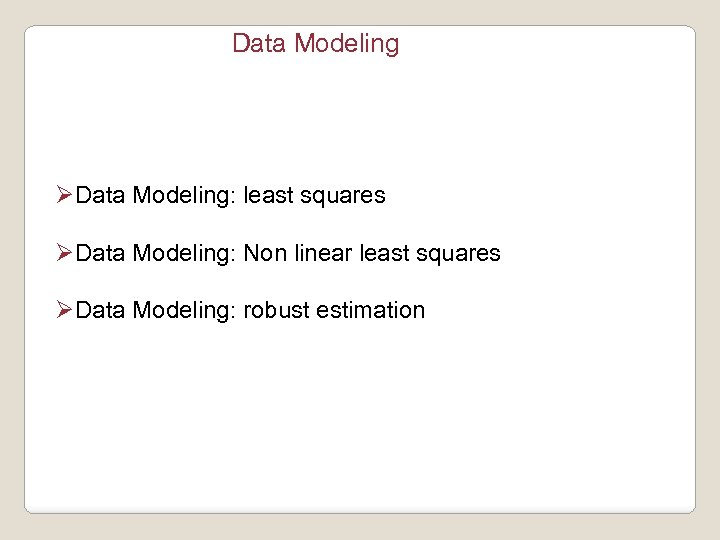Data Modeling ØData Modeling: least squares ØData Modeling: Non linear least squares ØData Modeling: robust estimation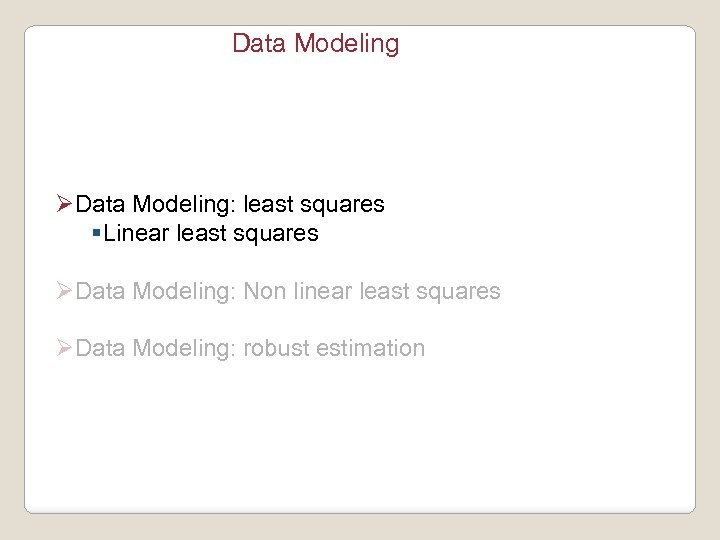Data Modeling ØData Modeling: least squares §Linear least squares ØData Modeling: Non linear least squares ØData Modeling: robust estimation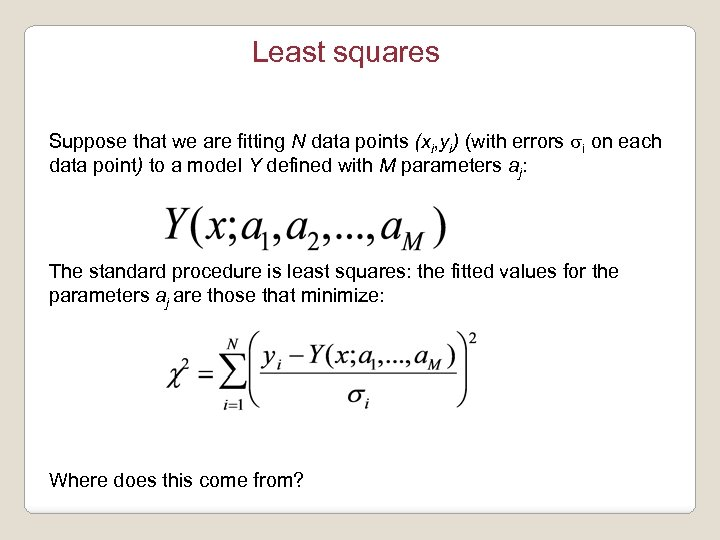Least squares Suppose that we are fitting N data points (xi, yi) (with errors si on each data point) to a model Y defined with M parameters aj: The standard procedure is least squares: the fitted values for the parameters aj are those that minimize: Where does this come from?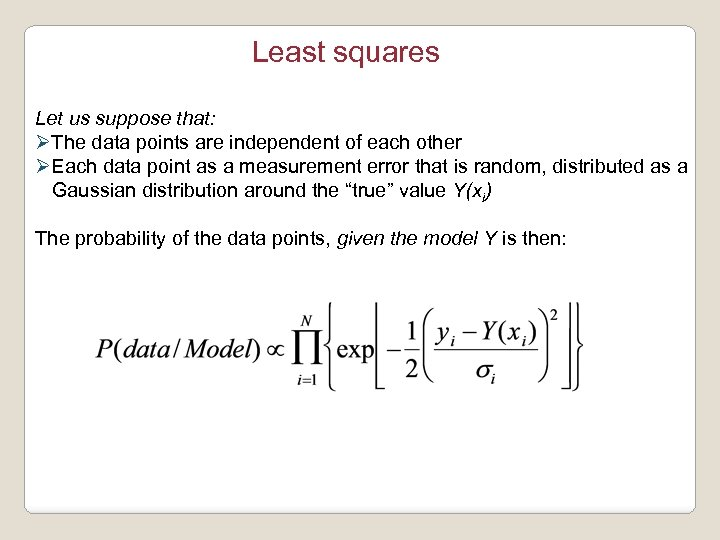Least squares Let us suppose that: ØThe data points are independent of each other ØEach data point as a measurement error that is random, distributed as a Gaussian distribution around the “true” value Y(xi) The probability of the data points, given the model Y is then: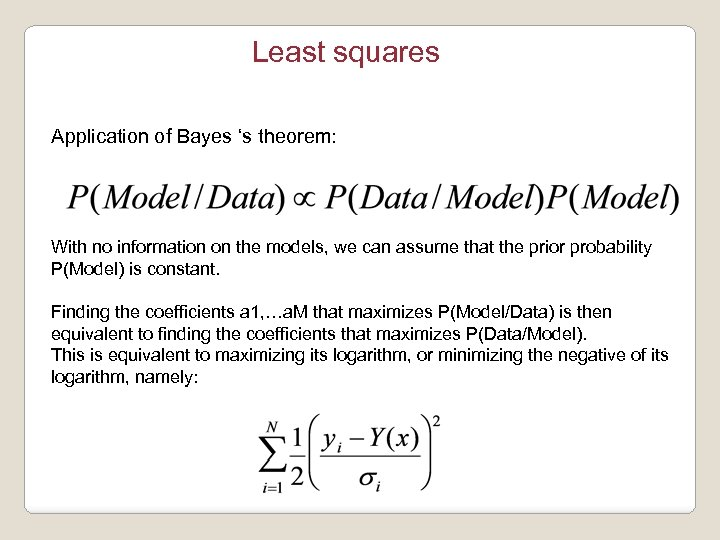Least squares Application of Bayes ‘s theorem: With no information on the models, we can assume that the prior probability P(Model) is constant. Finding the coefficients a 1, …a. M that maximizes P(Model/Data) is then equivalent to finding the coefficients that maximizes P(Data/Model). This is equivalent to maximizing its logarithm, or minimizing the negative of its logarithm, namely: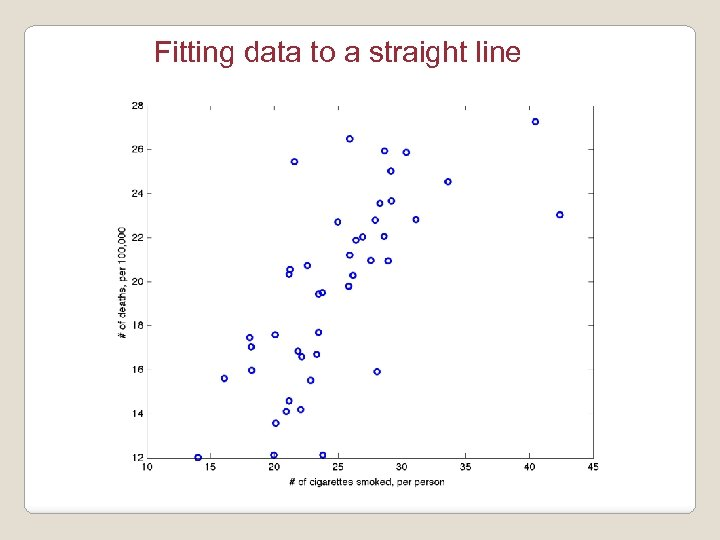Fitting data to a straight line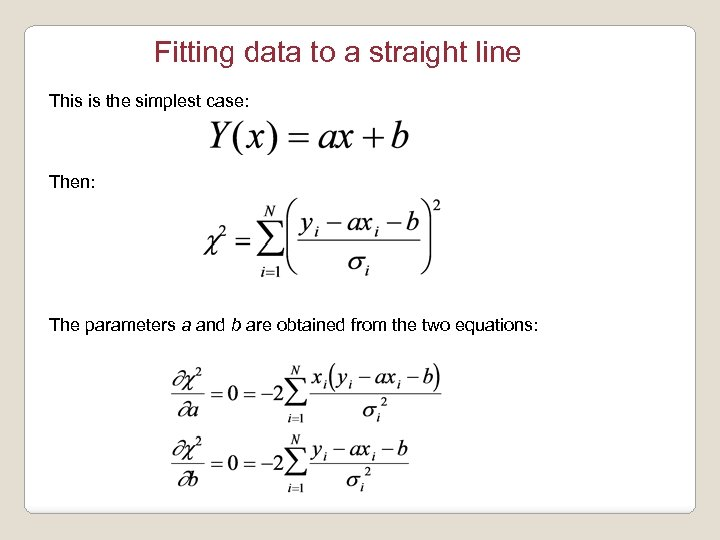Fitting data to a straight line This is the simplest case: Then: The parameters a and b are obtained from the two equations: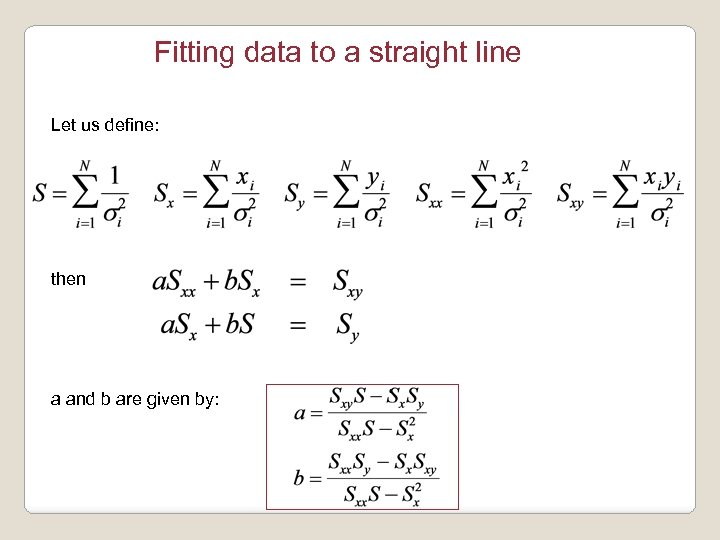Fitting data to a straight line Let us define: then a and b are given by: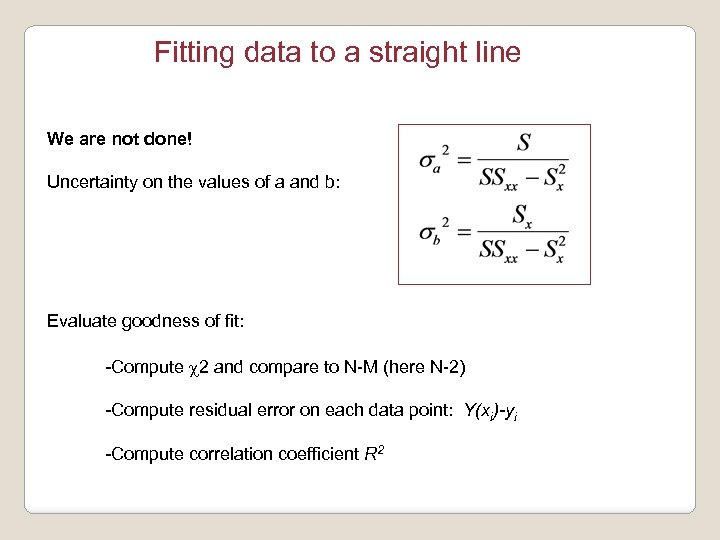Fitting data to a straight line We are not done! Uncertainty on the values of a and b: Evaluate goodness of fit: -Compute c 2 and compare to N-M (here N-2) -Compute residual error on each data point: Y(xi)-yi -Compute correlation coefficient R 2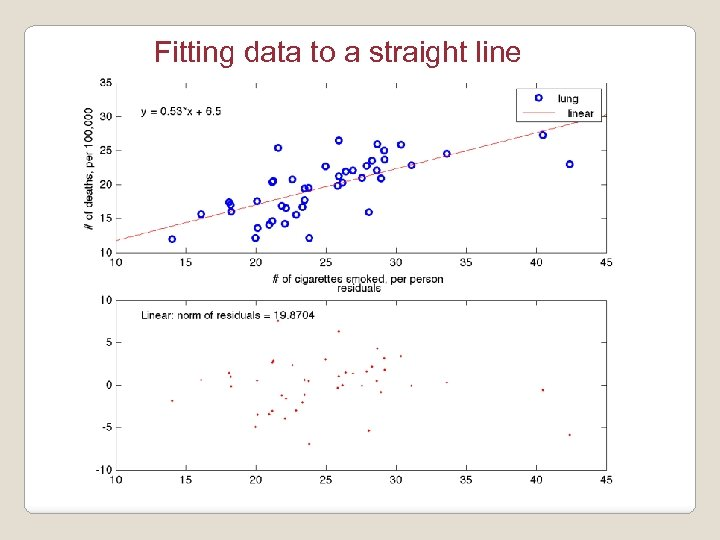Fitting data to a straight line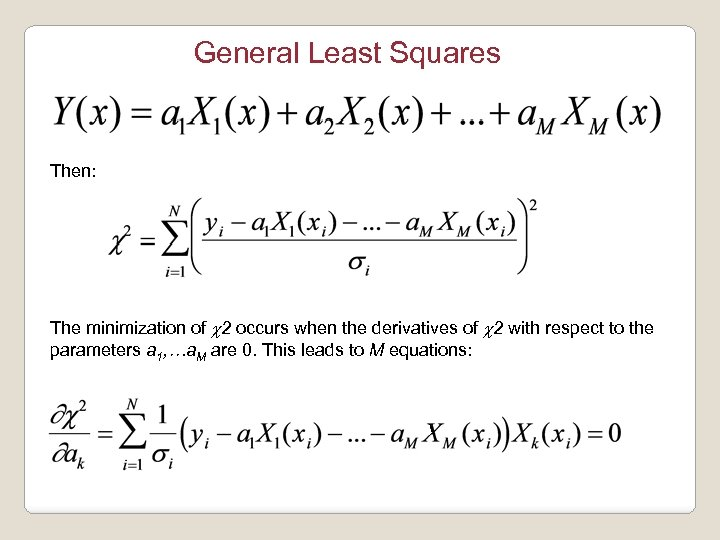General Least Squares Then: The minimization of c 2 occurs when the derivatives of c 2 with respect to the parameters a 1, …a. M are 0. This leads to M equations: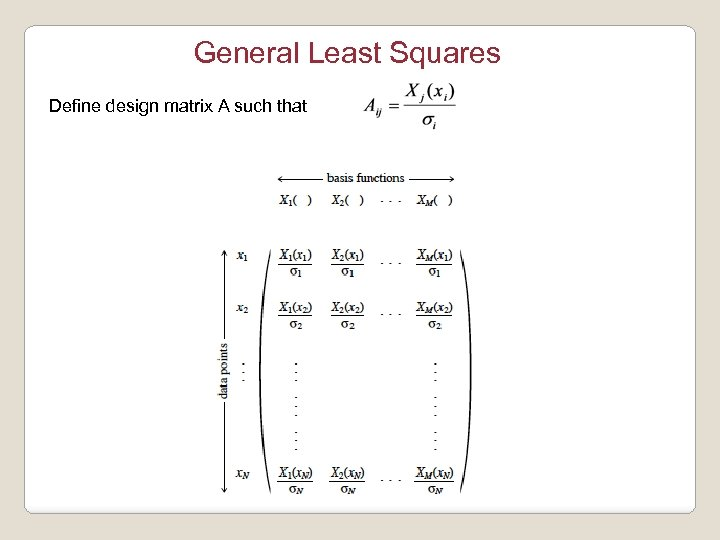General Least Squares Define design matrix A such that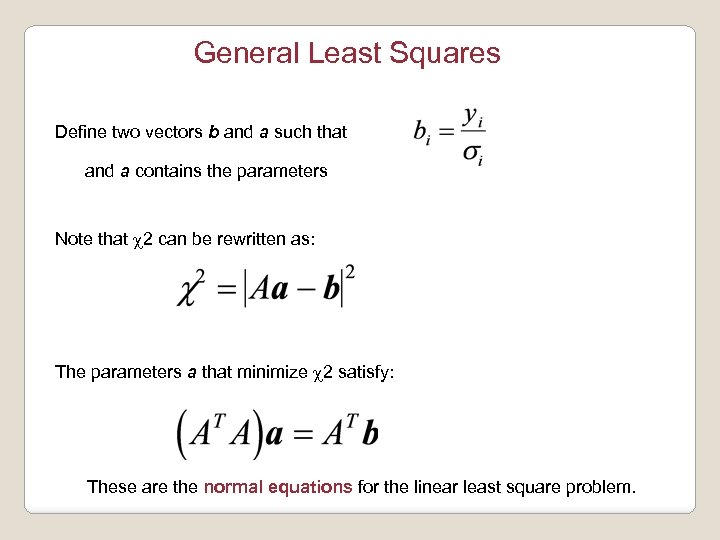General Least Squares Define two vectors b and a such that and a contains the parameters Note that c 2 can be rewritten as: The parameters a that minimize c 2 satisfy: These are the normal equations for the linear least square problem.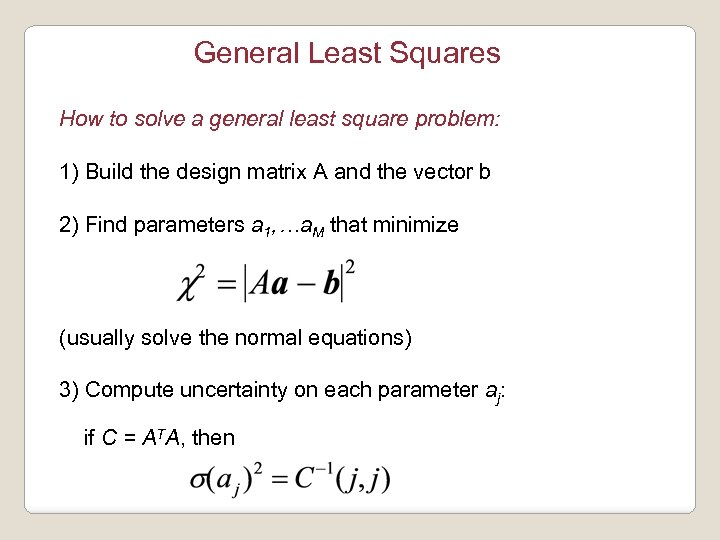General Least Squares How to solve a general least square problem: 1) Build the design matrix A and the vector b 2) Find parameters a 1, …a. M that minimize (usually solve the normal equations) 3) Compute uncertainty on each parameter aj: if C = ATA, thenData Modeling ØData Modeling: least squares ØData Modeling: Non linear least squares ØData Modeling: robust estimation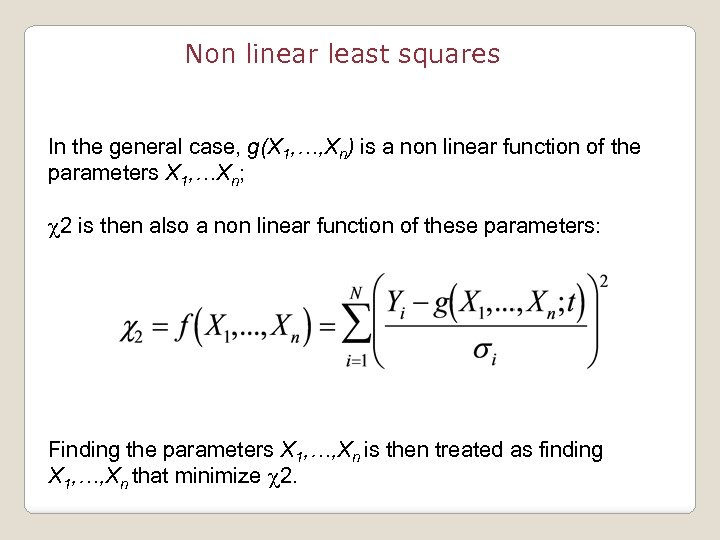Non linear least squares In the general case, g(X 1, …, Xn) is a non linear function of the parameters X 1, …Xn; c 2 is then also a non linear function of these parameters: Finding the parameters X 1, …, Xn is then treated as finding X 1, …, Xn that minimize c 2.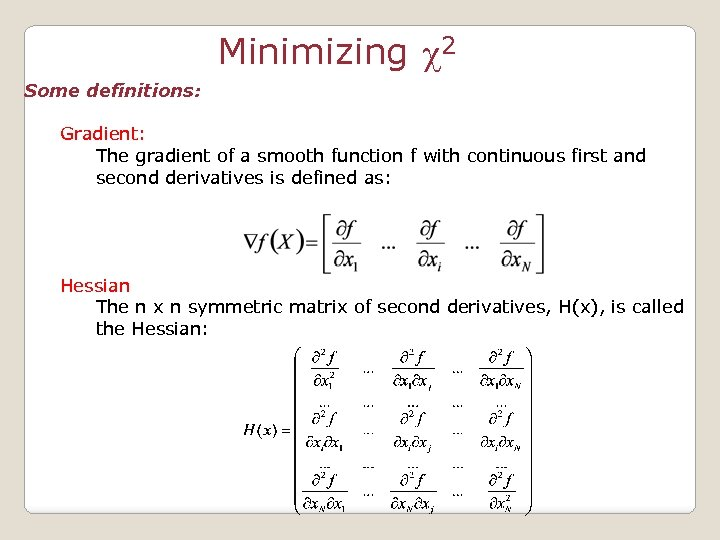Minimizing c 2 Some definitions: Gradient: The gradient of a smooth function f with continuous first and second derivatives is defined as: Hessian The n x n symmetric matrix of second derivatives, H(x), is called the Hessian: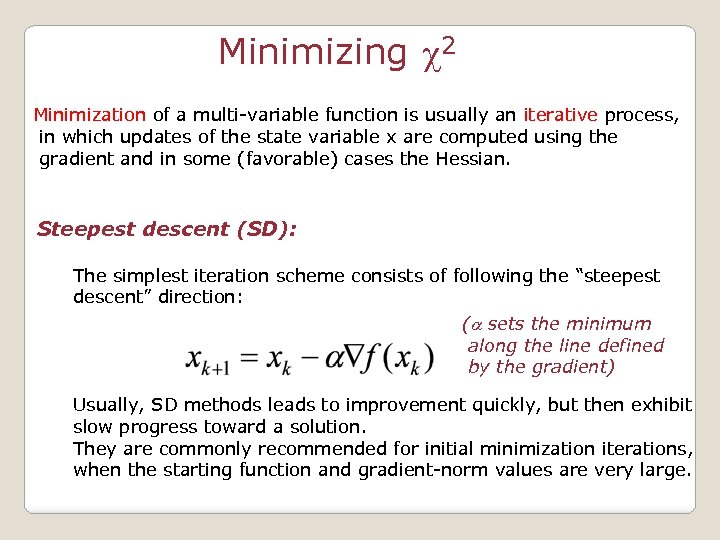Minimizing c 2 Minimization of a multi-variable function is usually an iterative process, in which updates of the state variable x are computed using the gradient and in some (favorable) cases the Hessian. Steepest descent (SD): The simplest iteration scheme consists of following the “steepest descent” direction: (a sets the minimum along the line defined by the gradient) Usually, SD methods leads to improvement quickly, but then exhibit slow progress toward a solution. They are commonly recommended for initial minimization iterations, when the starting function and gradient-norm values are very large.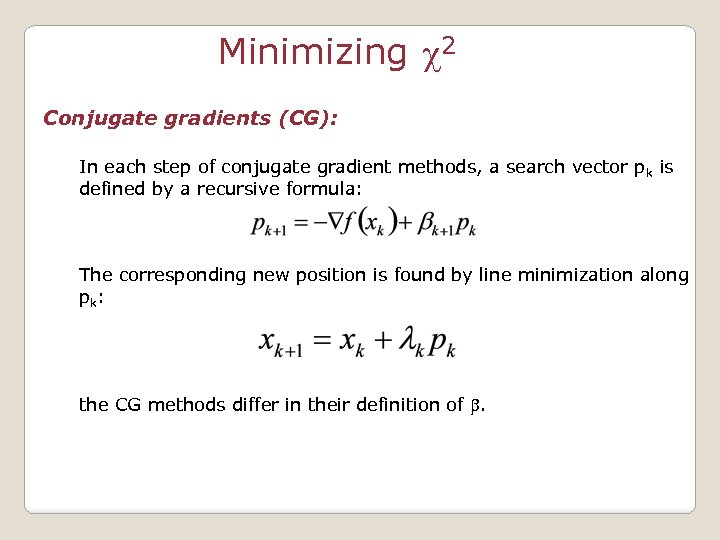Minimizing c 2 Conjugate gradients (CG): In each step of conjugate gradient methods, a search vector p k is defined by a recursive formula: The corresponding new position is found by line minimization along pk: the CG methods differ in their definition of .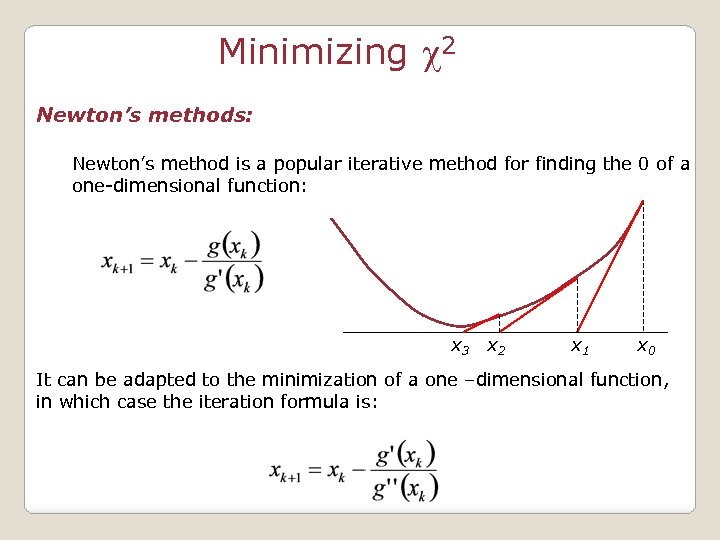Minimizing c 2 Newton’s methods: Newton’s method is a popular iterative method for finding the 0 of a one-dimensional function: x 3 x 2 x 1 x 0 It can be adapted to the minimization of a one –dimensional function, in which case the iteration formula is: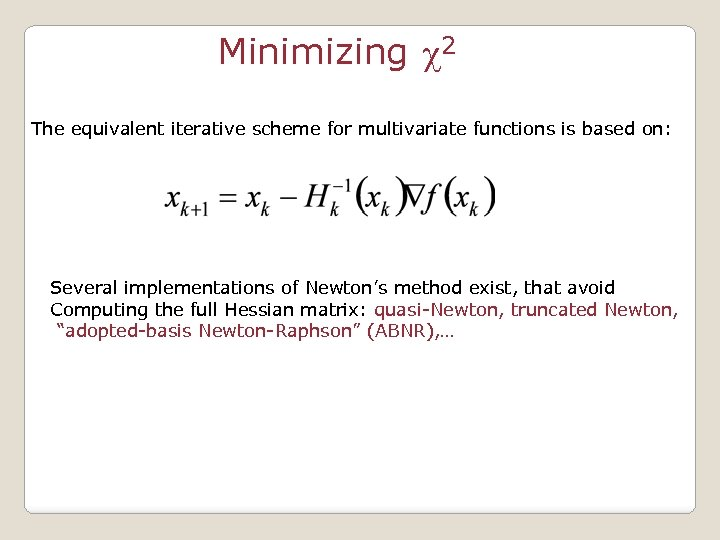Minimizing c 2 The equivalent iterative scheme for multivariate functions is based on: Several implementations of Newton’s method exist, that avoid Computing the full Hessian matrix: quasi-Newton, truncated Newton, “adopted-basis Newton-Raphson” (ABNR), …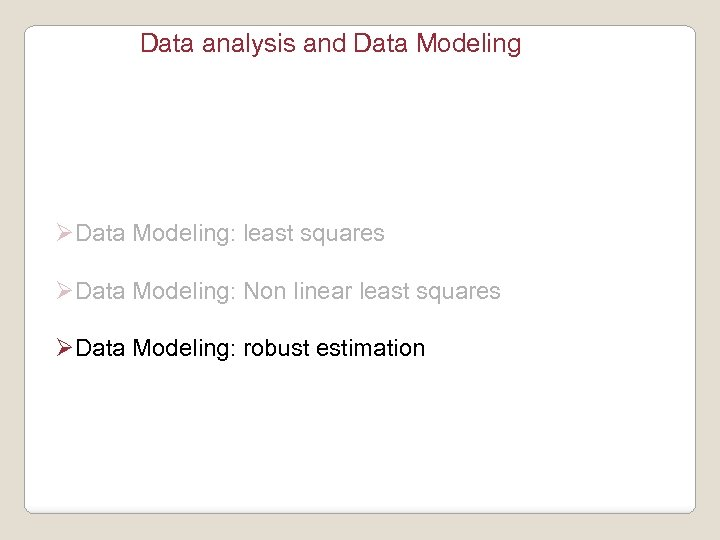Data analysis and Data Modeling ØData Modeling: least squares ØData Modeling: Non linear least squares ØData Modeling: robust estimation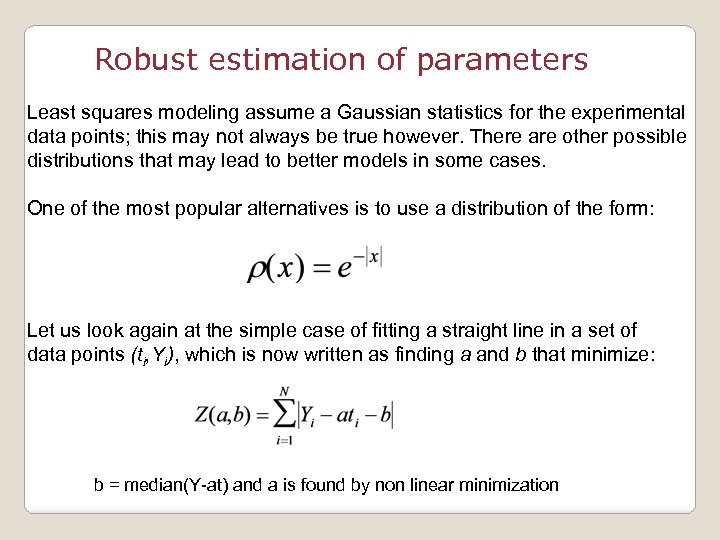Robust estimation of parameters Least squares modeling assume a Gaussian statistics for the experimental data points; this may not always be true however. There are other possible distributions that may lead to better models in some cases. One of the most popular alternatives is to use a distribution of the form: Let us look again at the simple case of fitting a straight line in a set of data points (ti, Yi), which is now written as finding a and b that minimize: b = median(Y-at) and a is found by non linear minimization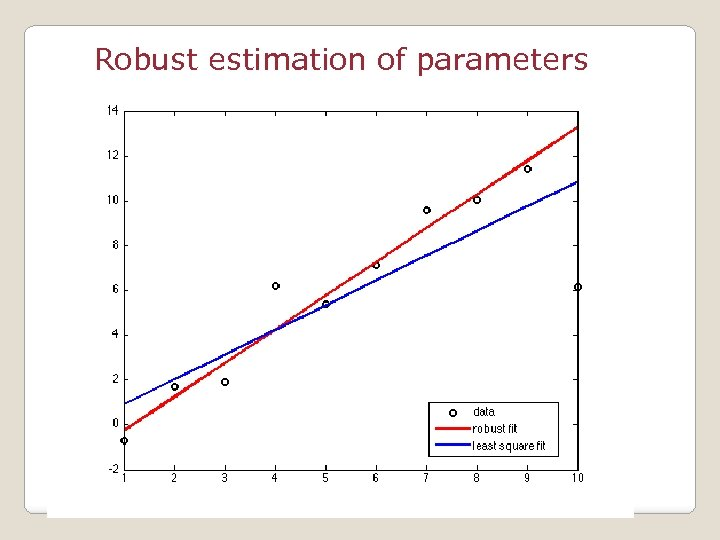Robust estimation of parameters